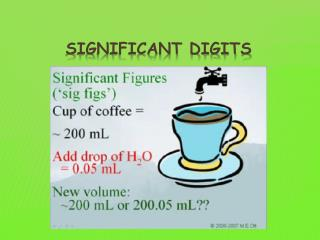DownloadDownload PresentationSignificant digits

# Significant digits

Download Presentation## Significant digits

- - - - - - - - - - - - - - - - - - - - - - - - - - - E N D - - - - - - - - - - - - - - - - - - - - - - - - - - -
##### Presentation Transcript

1. Significant digits

2. 1. Non-zero digits are ALWAYS significant. Example- 123456789 2. Zeros that are between other significant digits are ALWAYS significant. Example- 3004  3. Final zeros that occur before or after the decimal are ALWAYS significant. Example- 78.00 4. Zeros used for spacing the decimal are NOT significant. Example- 0.003 RULES FOR SIGNIFICANT DIGITS

3. EXAMPLES

4. 2804 0.00305 2.84 30.24 0.0029 6.89 4.6 X 104 0.001 75.00 6.0 50000 123.056 360 100.04 HOW MANY SIGNIFICANT DIGITS?

5. When you have numbers to add/subtract you will sometimes need your answer to have the correct number of significant digits. Here is the rule: Look at all of the numbers to add/subtract and find the number with the LEAST number of sig. digs. AFTER the decimal. This is how many sig. digs. should be after the decimal in your answer. Example - 24.686 2.343 3.21 30.239 ANSWER = 30.24 ADDING & SUBTRACTING

6. When you have numbers to multiply/divide you will sometimes need your answer to have the correct number of significant digits. Here is the rule: Look at all of the numbers to multiply/divide and find the number with the LEAST number of sig. digs. This is how many sig. digs. that you should have in your answer. Example - 3.22 x 2.1 = 6.762 ANSWER = 6.8 MULTIPLYING & DIVIDING

7. 1.2 + 1.26 = • 2.46 • Final Answer: 2.5 • 2.476-1.72 = • 0.756 • Final Answer: 0.76 • 10.72694321 – 10.1 = • 0.62694321 • Final Answer: 0.6 EXAMPLE PROBLEMS FOR +/-

8. 1.53 x 2.5 • 3.825 • Final Answer: 3.8 • 10.0 / 5.0 = • 2 • Final Answer: 2.0 • 10.2 x 1000 • 102,000 • Final Answer: 10.2 x 10³ EXAMPLE PROBLEMS FOR X/÷